Documentation

### This is machine translation

Mouseover text to see original. Click the button below to return to the English version of the page.

## Voice Activity Detection in Noise Using Deep Learning

This example shows how to detect regions of speech in a low signal-to-noise environment using deep learning. The example uses the Speech Commands Dataset to train a Bidirectional Long Short-Term Memory (BiLSTM) network to detect voice activity.

### Introduction

Voice activity detection is an essential component of many audio systems, such as automatic speech recognition and speaker recognition. Voice activity detection can be especially challenging in low signal-to-noise (SNR) situations, where speech is obstructed by noise.

This example uses long short-term memory (LSTM) networks, which are a type of recurrent neural network (RNN) well-suited to study sequence and time-series data. An LSTM network can learn long-term dependencies between time steps of a sequence. An LSTM layer (`lstmLayer`) can look at the time sequence in the forward direction, while a bidirectional LSTM layer (`bilstmLayer`) can look at the time sequence in both forward and backward directions. This example uses a bidirectional LSTM layer.

This example trains a voice activity detection bidirectional LSTM network with feature sequences of spectral characteristics and a harmonic ratio metric.

To run the example, you must first download the data set. If you do not want to download the data set or train the network, then you can load a pretrained network by opening this example in MATLAB® and typing `load("speechDetectNet.mat")` at the command line.

### Example Summary

The example goes through the following steps:

Training:

1. Create an `audioDatastore` that points to the audio speech files used to train the LSTM network.

2. Create a training signal consisting of speech segments separated by segments of silence of varying durations.

3. Corrupt the speech-plus-silence signal with washing machine noise (SNR = -10 dB).

4. Extract feature sequences consisting of spectral characteristics and harmonic ratio from the noisy signal.

5. Train the LSTM network using the feature sequences to identify regions of voice activity.

Prediction:

1. Create an `audioDatastore` of speech files used to test the trained network, and create a test signal consisting of speech separated by segments of silence.

2. Corrupt the test signal with washing machine noise (SNR = -10 dB).

3. Extract feature sequences from the noisy test signal.

4. Identify regions of voice activity by passing the test features through the trained network.

5. Compare the network's accuracy to the voice activity baseline from the signal-plus-silence test signal.

Here is a sketch of the training process.Here is a sketch of the prediction process. You use the trained network to make predictions.### Load Speech Commands Data Set

Download the data set from https://download.tensorflow.org/data/speech_commands_v0.01.tar.gz and extract the downloaded file. Set `datafolder` to the location of the data. Use `audioDatastore` to create a datastore that contains the file names.

```datafolder = PathToDatabase; ads = audioDatastore(datafolder,"Includesubfolders",true); ```

The dataset contains background noise files that are not used in this example. Use `subset` to create a new datastore that does not have the background noise files.

```indices = cellfun(@(c)~contains(c,"_background_noise_"),ads.Files); ads = subset(ads,indices); ```

### Split Data into Training and Validation

The data set folder contains text files that lists which audio files should be in the validation set and which audio files should be in the test set. These predefined validation and test sets do not contain utterances of the same word by the same person, so it is better to use these predefined sets than to select a random subset of the whole data set. Use the supporting function `splitData` to split the datastore into training and validation sets based on the list of validation and test files.

```[adsTrain,adsValidation] = splitData(ads,datafolder); ```

Shuffle the order of files in the datastores.

```adsTrain = shuffle(adsTrain); adsValidation = shuffle(adsValidation); ```

### Create Speech-Plus-Silence Training Signal

Read the contents of an audio file using `read`. Get the sample rate from the `info` struct.

```[data,info] = read(adsTrain); Fs = info.SampleRate; ```

Listen to the audio signal using the sound command.

```sound(data,Fs) ```

Plot the audio signal.

```timeVector = (1/Fs) * (0:numel(data)-1); plot(timeVector,data) ylabel("Amplitude") xlabel("Time (s)") title("Sample Audio") grid on ```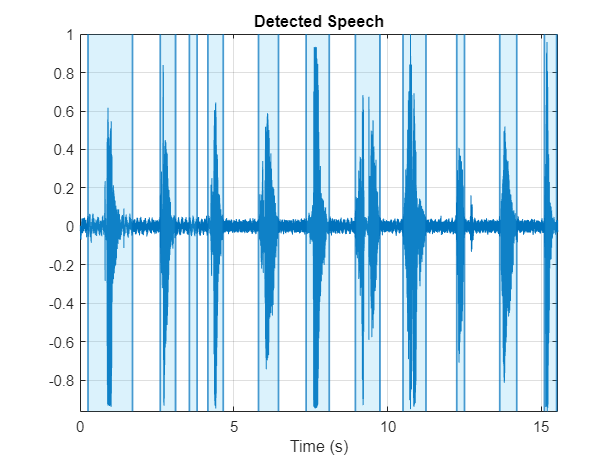The signal has non-speech portions (silence, background noise, etc) that do not contain useful speech information. This example removes silence using a simple thresholding approach identical to the one used in Classify Gender Using Long Short-Term Memory Networks.

Extract the useful portion of data. Plot the new audio signal and listen to it using the `sound` command.

```segments = HelperGetSpeechSegments(data,Fs); speechData = segments{1}; figure timeVector = (1/Fs) * (0:numel(speechData)-1); plot(timeVector,speechData) ylabel("Amplitude") xlabel("Time (s)") title("Sample Audio") grid on ```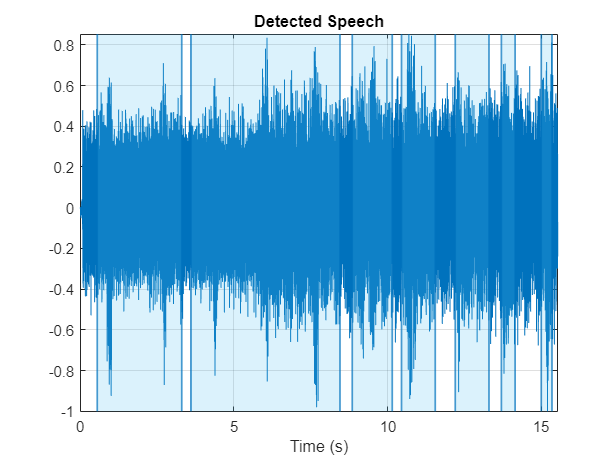Create a 1000-second training signal by combining multiple speech files from the training dataset. Use `HelperGetSpeechSegments` to remove unwanted portions of each file. Insert a random period of silence between speech segments.

Preallocate the training signal.

```duration = 1000*Fs; audioTraining = zeros(duration,1); ```

Preallocate the voice activity training mask. Values of 1 in the mask correspond to samples located in areas with voice activity. Values of 0 correspond to areas with no voice activity.

```maskTraining = zeros(duration,1); ```

Specify a maximum silence segment duration of 2 seconds.

```maxSilenceSegment = 2; ```

Construct the training signal by calling `read` on the datastore in a loop.

```numSamples = 1; while numSamples < duration data = read(adsTrain); % Remove non-voice areas from the segment data = HelperGetSpeechSegments(data,Fs); data = data{1}; % Write speech segment to training signal audioTraining(numSamples:numSamples+numel(data)-1) = data; % Set VAD baseline maskTraining(numSamples:numSamples+numel(data)-1) = true; % Random silence period numSilenceSamples = randi(maxSilenceSegment * Fs,1,1); numSamples = numSamples + numel(data) + numSilenceSamples; end ```

Visualize a 10-second portion of the training signal. Plot the baseline voice activity mask.

```figure range = 1:10*Fs; plot((1/Fs)*(range-1),audioTraining(range)); hold on plot((1/Fs)*(range-1),maskTraining(range)); grid on lines = findall(gcf,"Type","Line"); lines(1).LineWidth = 2; xlabel("Time (s)") legend("Signal","Speech Area") title("Training Signal (first 10 seconds)"); ```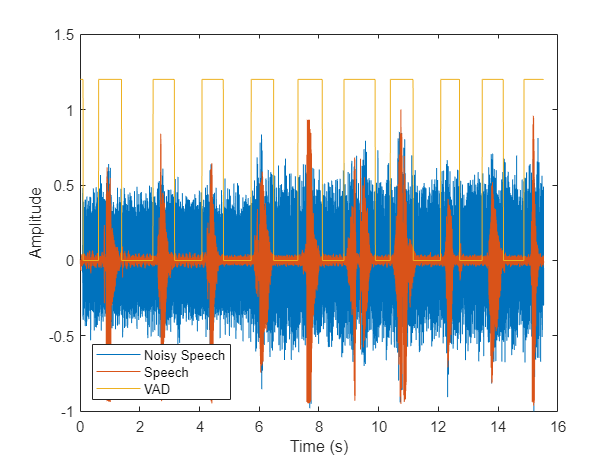Listen to the first 10 seconds of the training signal.

```sound(audioTraining(range),Fs); ```

### Add Noise to the Training Signal

Corrupt the training signal with washing machine noise by adding washing machine noise to the speech signal such that the signal-to-noise ratio is -10 dB.

Read 8 kHz noise and convert it to 16 kHz.

```noise = audioread("WashingMachine-16-8-mono-1000secs.wav"); noise = resample(noise,2,1); ```

Corrupt training signal with noise.

```audioTraining = audioTraining(1:numel(noise)); SNR = -10; noise = 10^(-SNR/20) * noise * norm(audioTraining) / norm(noise); audioTrainingNoisy = audioTraining + noise; audioTrainingNoisy = audioTrainingNoisy / max(abs(audioTrainingNoisy)); ```

Visualize a 10-second portion of the noisy training signal. Plot the baseline voice activity mask.

```figure plot((1/Fs)*(range-1),audioTrainingNoisy(range)); hold on plot((1/Fs)*(range-1),maskTraining(range)); grid on lines = findall(gcf,"Type","Line"); lines(1).LineWidth = 2; xlabel("Time (s)") legend("Noisy Signal","Speech Area") title("Training Signal (first 10 seconds)"); ```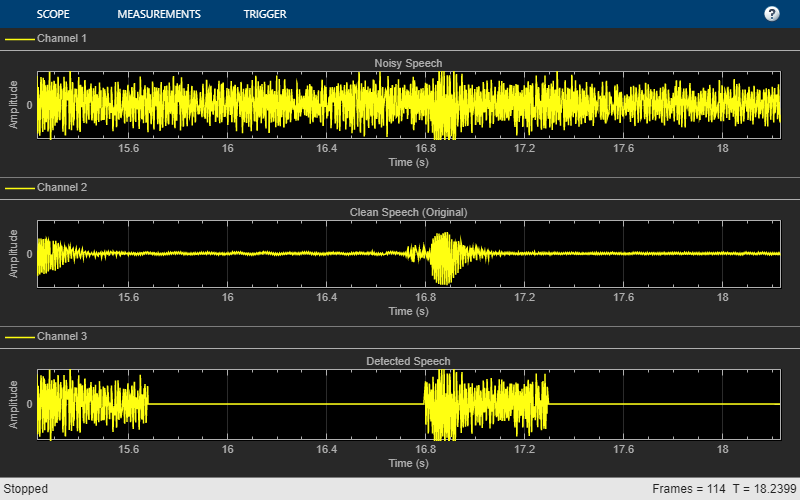Listen to the first 10 seconds of the noisy training signal.

```sound(audioTrainingNoisy(range),Fs) ```

Note that you obtained the baseline voice activity mask using the noiseless speech-plus-silence signal. Verify that using `HelperGetSpeechSegments` on the noise-corrupted signal does not yield good results.

```[~,noisyMask] = HelperGetSpeechSegments(audioTrainingNoisy,Fs); ```

Visualize a 10-second portion of the noisy training signal. Plot the voice activity mask obtained by analyzing the noisy signal.

```figure plot((1/Fs)*(range-1),audioTrainingNoisy(range)); hold on plot((1/Fs)*(range-1),noisyMask(range)); grid on lines = findall(gcf,"Type","Line"); lines(1).LineWidth = 2; xlabel("Time (s)") legend("Noisy Signal","Mask from Noisy Signal") title("Training Signal (first 10 seconds)"); ```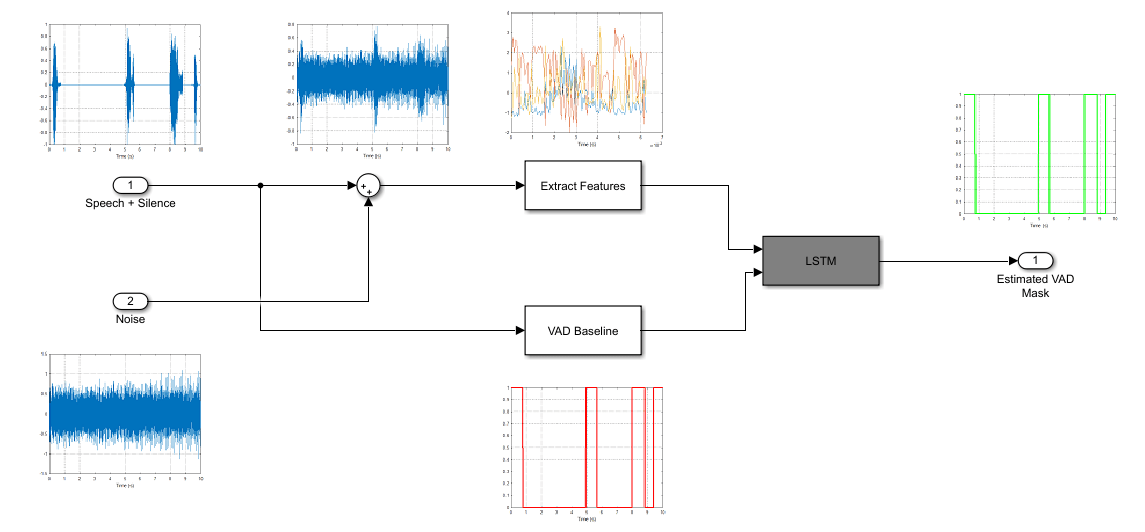### Create Speech-Plus-Silence Validation Signal

Create a 200-second noisy speech signal to validate the trained network. Use the validation datastore. Note that the validation and training datastores have different speakers.

Preallocate the validation signal and the validation mask. You will use this mask to assess the accuracy of the trained network.

```duration = 200*Fs; audioValidation = zeros(duration,1); maskValidation = zeros(duration,1); ```

Construct the validation signal by calling `read` on the datastore in a loop.

```numSamples = 1; while numSamples < duration data = read(adsValidation); % Remove non-voice areas from the segment data = HelperGetSpeechSegments(data,Fs); data = data{1}; % Write speech segment to training signal audioValidation(numSamples:numSamples+numel(data)-1) = data; maskValidation(numSamples:numSamples+numel(data)-1) = true; % Random silence period numSilenceSamples = randi(maxSilenceSegment*Fs,1,1); numSamples = numSamples + numel(data) + numSilenceSamples; end ```

Corrupt the validation signal with washing machine noise by adding washing machine noise to the speech signal such that the signal-to-noise ratio is -10 dB. Use a different noise file for the validation signal than you did for the training signal.

```noise = audioread("WashingMachine-16-8-mono-200secs.wav"); noise = resample(noise,2,1); audioValidation = audioValidation(1:numel(noise)); noise = 10^(-SNR/20) * noise * norm(audioValidation) / norm(noise); audioValidationNoisy = audioValidation + noise; audioValidationNoisy = audioValidationNoisy / max(abs(audioValidationNoisy)); ```

### Extract Training Features

This example trains the LSTM network using the following features:

Define system parameters.

```WindowLength = 256; params.WindowLength = WindowLength; params.Window = hann(WindowLength,"Periodic"); params.OverlapLength = 128; params.FFTLength = 256; params.Range = [0, Fs/2]; params.SpectrumType = "Power"; ```

Compute the power spectrum of the training signal.

```[~,frequencyVector,~,S] = spectrogram(audioTrainingNoisy,hann(WindowLength,"Periodic"),128,WindowLength,Fs,"power" ,"onesided"); ```

Extract the features and then concatenate them.

```SCentroid = spectralCentroid(S,frequencyVector); SCrest = spectralCrest(S,frequencyVector); Sentropy = spectralEntropy(S,frequencyVector); SFlux = spectralFlux(S,frequencyVector); SKurtosis = spectralKurtosis(S,frequencyVector); SRolloffPoint = spectralRolloffPoint(S,frequencyVector); SSkewness = spectralSkewness(S,frequencyVector); SSlope = spectralSlope(S,frequencyVector); hr = harmonicRatio(audioTrainingNoisy,Fs,"Window",params.Window,"OverlapLength",params.OverlapLength); featuresTraining = [SCentroid SCrest Sentropy SFlux SKurtosis SRolloffPoint SSkewness SSlope hr]; ```

Display the dimensions of the features matrix. The first dimension corresponds to the number of windows the signal was broken into (it depends on the window length and the overlap length). The second dimension is the number of features used in this example.

```[numWindows,numFeatures] = size(featuresTraining) ```
```numWindows = 124999 numFeatures = 9 ```

In classification applications, it is a good practice to normalize all features to have zero mean and unity standard deviation.

Compute the mean and standard deviation for each coefficient, and use them to normalize the data.

```M = mean(featuresTraining,1); S = std(featuresTraining,[],1); featuresTraining = (featuresTraining - M) ./ S; ```

Extract the features from the validation signal using the same process. The helper function `getSpeechActivityFeatures` encapsulates the feature extraction and normalization steps.

```featuresValidation = getSpeechActivityFeatures(audioValidationNoisy,Fs,params); ```

Each feature corresponds to 128 samples of data (the hop length). For each hop, set the expected voice/no voice value to the mode of the baseline mask values corresponding to those 128 samples. Convert the voice/no voice mask to categorical.

```hopLength = (WindowLength - params.OverlapLength); range = (hopLength) * (1:size(featuresTraining,1)) + hopLength; maskMode = zeros(size(range)); for index = 1:numel(range) maskMode(index) = mode(maskTraining( (index-1)*hopLength+1:(index-1)*hopLength+WindowLength )); end maskTraining = maskMode.'; maskTrainingCat = categorical(maskTraining); ```

Do the same for the validation mask.

```range = (hopLength) * (1:size(featuresValidation,1)) + hopLength; maskMode = zeros(size(range)); for index = 1:numel(range) maskMode(index) = mode(maskValidation( (index-1)*hopLength+1:(index-1)*hopLength+WindowLength )); end maskValidation = maskMode.'; maskValidationCat = categorical(maskValidation); ```

Split the training features and the mask into sequences of length 800, with 75% overlap between consecutive sequences.

```sequenceLength = 800; trainFeatureCell = {}; trainLabelCell = {}; index = 1; while index < size(featuresTraining,1) - sequenceLength trainFeatureCell{end+1} = featuresTraining(index:index+sequenceLength-1,:).'; %#ok trainLabelCell{end+1} = maskTrainingCat(index:index+sequenceLength-1).'; %#ok index = index + round(sequenceLength/4); end ```

### Define the LSTM Network Architecture

LSTM networks can learn long-term dependencies between time steps of sequence data. This example uses the bidirectional LSTM layer `bilstmLayer` to look at the sequence in both forward and backward directions.

Specify the input size to be sequences of length `9` (the number of features). Specify a hidden bidirectional LSTM layer with an output size of 200 and output a sequence. This command instructs the bidirectional LSTM layer to map the input time series into 200 features that are passed to the next layer. Then, specify a bidirectional LSTM layer with an output size of 200 and output the last element of the sequence. This command instructs the bidirectional LSTM layer to map its input into 200 features and then prepares the output for the fully connected layer. Finally, specify two classes by including a fully connected layer of size `2`, followed by a softmax layer and a classification layer.

```layers = [ ... sequenceInputLayer( size(featuresValidation,2) ) bilstmLayer(200,"OutputMode","sequence") bilstmLayer(200,"OutputMode","sequence") fullyConnectedLayer(2) softmaxLayer classificationLayer ]; ```

Next, specify the training options for the classifier. Set `MaxEpochs` to `20` so that the network makes 20 passes through the training data. Set `MiniBatchSize` to `64` so that the network looks at 64 training signals at a time. Set `Plots` to `"training-progress"` to generate plots that show the training progress as the number of iterations increases. Set `Verbose` to `false` to disable printing the table output that corresponds to the data shown in the plot. Set `Shuffle` to `"every-epoch"` to shuffle the training sequence at the beginning of each epoch. Set `LearnRateSchedule` to `"piecewise"` to decrease the learning rate by a specified factor (0.1) every time a certain number of epochs (10) has passed. Set `ValidationData` to the validation predictors and targets.

This example uses the adaptive moment estimation (ADAM) solver. ADAM performs better with recurrent neural networks (RNNs) like LSTMs than the default stochastic gradient descent with momentum (SGDM) solver.

```maxEpochs = 20; miniBatchSize = 64; options = trainingOptions("adam", ... "MaxEpochs",maxEpochs, ... "MiniBatchSize",miniBatchSize, ... "Shuffle","every-epoch",... "Verbose",0, ... "SequenceLength",sequenceLength,... "ValidationFrequency",floor(numel(trainFeatureCell)/miniBatchSize),... "ValidationData",{featuresValidation.',maskValidationCat.'},... "Plots","training-progress",... "LearnRateSchedule","piecewise",... "LearnRateDropFactor",0.1, ... "LearnRateDropPeriod",10); ```

### Train the LSTM Network

Train the LSTM network with the specified training options and layer architecture using `trainNetwork`. Because the training set is large, the training process can take several minutes.

```doTraining = true; if doTraining [speechDetectNet,info] = trainNetwork(trainFeatureCell,trainLabelCell,layers,options); fprintf("Validation accuracy: %f percent.\n" , info.ValidationAccuracy(end)); else load speechDetectNet end ```
```Validation accuracy: 90.734375 percent. ```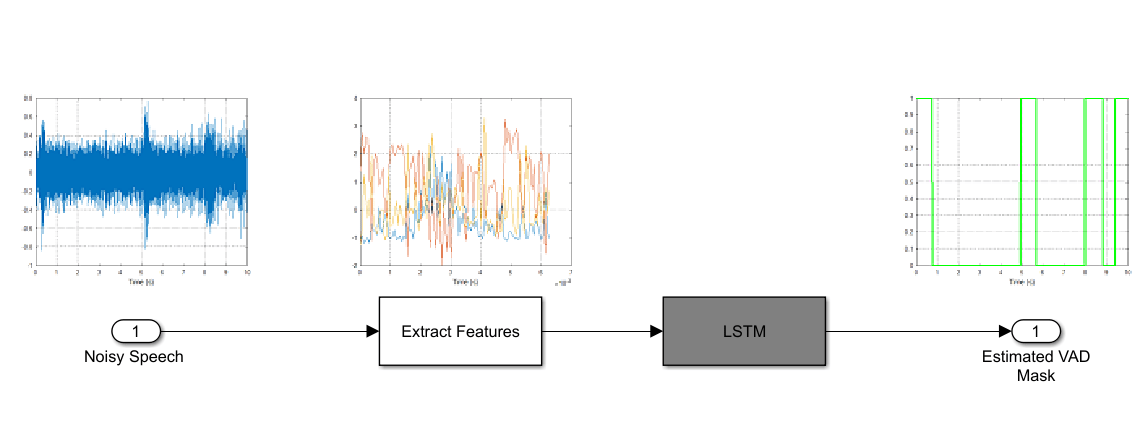### Use Trained Network to Detect Voice Activity

Estimate voice activity in the validation signal using the trained network. Convert the estimated VAD mask from categorical to double.

```EstimatedVADMask = classify(speechDetectNet,featuresValidation.'); EstimatedVADMask = double(EstimatedVADMask); EstimatedVADMask = EstimatedVADMask.' - 1; ```

Calculate and plot the validation confusion matrix from the vectors of actual and estimated labels.

```figure cm = confusionchart(maskValidation,EstimatedVADMask,"title","Validation Accuracy"); cm.ColumnSummary = "column-normalized"; cm.RowSummary = "row-normalized"; ```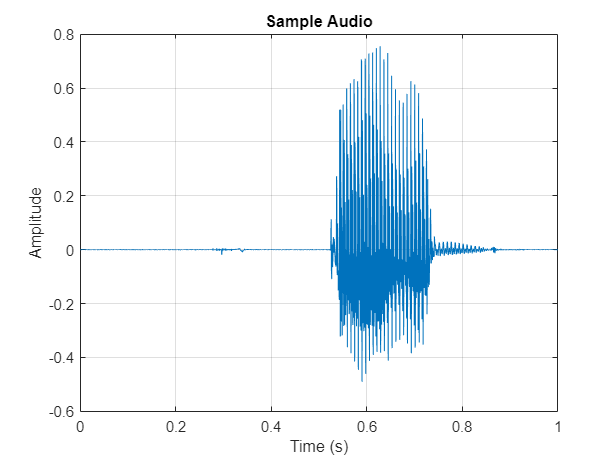Each mask value corresponds to 128 samples (the hop length). Repeat the mask values to make a mask of the same length as the validation audio signal. Do the same for the baseline mask.

```EstimatedVADMask = repmat(EstimatedVADMask,1,hopLength); EstimatedVADMask = reshape(EstimatedVADMask.',numel(EstimatedVADMask),1); ActualVADMask = repmat(maskValidation,1,hopLength); ActualVADMask = reshape(ActualVADMask.',numel(ActualVADMask),1); ```

Isolate the regions of estimated voice activity by setting the no-voice regions to `NaN`.

```audioEst = audioValidationNoisy; audioEst(EstimatedVADMask == 0) = NaN; audioAct = audioValidation; audioAct(ActualVADMask == 0) = NaN; ```

Visualize the estimated and actual areas of voice activity for a 50-second portion of the validation signal.

```figure range = 50*Fs:100*Fs; subplot(2,1,1) plot(range/Fs,[audioValidationNoisy(range),audioEst(range)]) title("Estimated Speech Region") legend("Signal","Speech Detected") grid on subplot(2,1,2) plot(range/Fs,[audioValidation(range),audioAct(range)]) title("Baseline") legend("Signal","Speech Detected") grid on xlabel("Time (s)") ```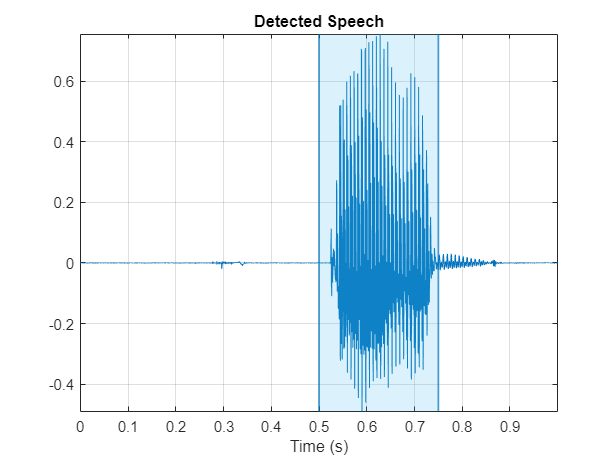##### Support평가판 신청